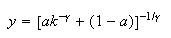### Basic Reminders about Production Function

#### Production Function With Complementary Factors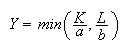(isoquant).

#### Production Function With Substituable Factors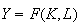,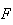continuous and twice derivable,,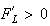,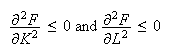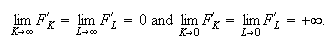#### Marginal Rate of Substitution

The differential of the production function: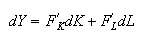along an isoquant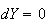and the Marginal Rate of Substitution (MRS) of Capital to Labor can be defined by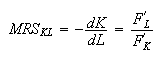#### Elasticity of substitution

Elasticity of the substitution of Capital to Labor: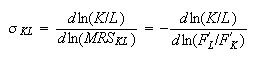Hypothesis of the remuneration of factors to their marginal productivity,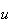nominal cost of capital,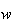nominal rate of wage,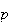level of prices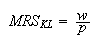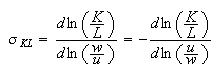#### Returns to Scale

. Constant if. Decreasing if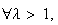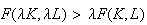. Increasing if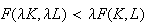Constant Returns to Scale

Euler theorem: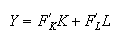that means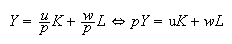Product Exhaustion Theorem

Zero-Profit

Reasoning per capita:per capita product (or mean productivity of labor)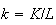per capita capital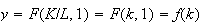Frontier of the prices of factors#### Cobb-Douglas Function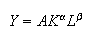with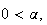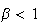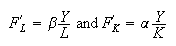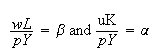If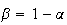, constant returns to scale. Then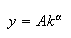and frontier of the prices of factors: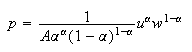#### C.E.S Function

Function of Constant Elasticity of Substitution (CES)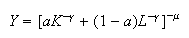with,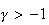,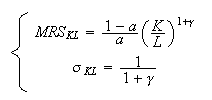Constant returns to scale if, increasing if, decreasing if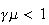.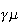is the scale elasticity. The per capita formulation of the CES function with constant returns to scale: